2019-04-03 14:09:32 qq_35789421 阅读数 684
• ###### MATLAB图像处理

全面系统的学习MATLAB在图像处理中的应用

20258 人正在学习 去看看 魏伟

Opencv中提供的inpaint函数可以实现图像修复操作，具体参数详细介绍如下：

<1>计算边界内部区域上p点一阶导数，以p点为中心选取一个小邻域，根据给定的q点像素及其在该点的梯度值，可以计算出p点一阶导数：

<2>计算p点新像素值，根据邻域作用差别性，引入权重函数,权重函数的计算通过距离参数与水平集参数获得，然后通过下式来计算新的p点像素值：

<3>对上述图像进行新像素值生成操作，先完成待修复区域边缘上的像素点，逐步收缩待修复区域的边界，直至完成整个区域的修复。

2020-01-17 22:46:34 qq_19409845 阅读数 105
• ###### MATLAB图像处理

全面系统的学习MATLAB在图像处理中的应用

20258 人正在学习 去看看 魏伟

# 深度学习应用发展

• 图像方面：图像识别、手机解锁、物体识别、图片修复、目标检测、医疗影像诊断。
• 自然语言处理：机器创作、个性化推荐、文本分类、翻译、智能音箱、自动纠错、情感分析。
• 数值预测、量化交易

# 神经网络是什么？

• 我们以房价预测的案例来说明一下，把房屋的面积作为神经网络的输入（我们称之为𝑥），通过一个节点（一个小圆圈），最终输出了价格（我们用𝑦表示）。其实这个小圆圈就是一个单独的神经元，就像人的大脑神经元一样。如果这是一个单神经元网络，不管规模大小，它正是通过把这些单个神经元叠加在一起来形成。如果你把这些神经元想象成单独的乐高积木，你就通过搭积木来完成一个更大的神经网络。
• 大脑是由处理信息的神经元细胞和连接神经元的细胞进行信息传递的突触构成的。 树突(Dendrites)从一个神经元接受电信号，信号在细胞核(Cell Body)处理后， 然后通过轴突(Axon)将处理的信号传递给下一个神经元。神经网络来源之人的思考
• 一个神经元可以看作是将一个或多个输入处理成一 个输出的计算单元。
• 通过多个神经元的传递，最终大脑会得到这个信息， 并可以对这个信息给出一个合适的反馈。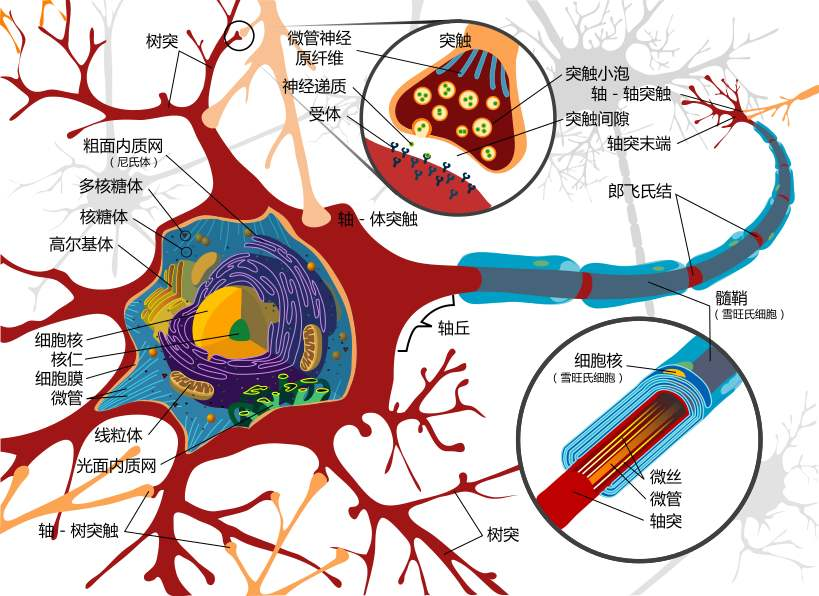## 感知机是什么！

• 感知器可以看作是根据权重来做出决定的一个设备/单元，只要我们可以给定一 个比较适合的权重以及阈值，那么感知器应该是能够对数据进行判断or分类预测。
• 感知器是一种模拟人的神经元的一种算法模型，是一种研究单个训练样本的二元分类器，是SVM和人工神 经网络(ANN, Artificial Neural Networks)的基础。
• 一个感知器接受几个二进制的输入，并产生一个二进制的输出，感知器模型的激活函数为（阶跃函数）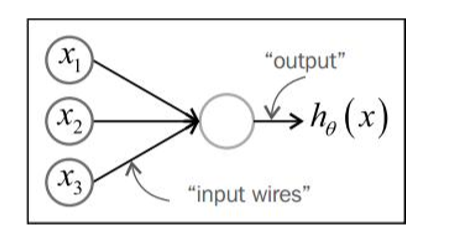$output= \begin{cases} 0，if&w\cdot x+b\leq0\\ 1，if&w\cdot x+b>0 \end{cases}$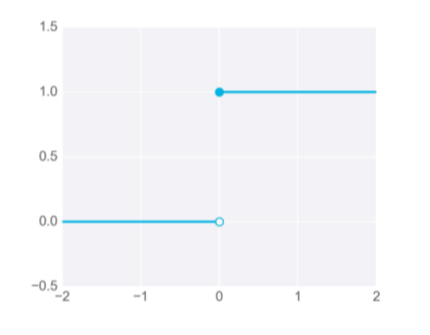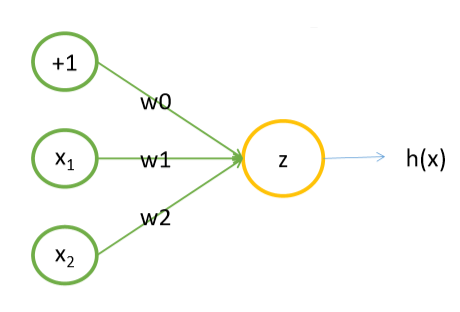• 单个神经元完成逻辑与（and）功能

$x_1,x_2\in\{0,1\}\\y=x_1 \quad and\quad x_2\\w=(-3,2,2)$$h_w(z)=h(-3\cdot1+2\cdot x_1+2\cdot x_2)=\begin{cases}0,z<0\\1,z\geq0\end{cases}$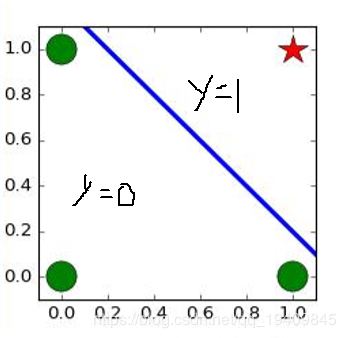$x_1$ $x_2$ $h_\theta(x)$
0 0 0
0 1 0
1 0 0
1 1 1
• 单个神经元完成逻辑或（or）功能

$x_1,x_2\in\{0,1\}\\y=x_1 \quad or\quad x_2\\w=(-1,2,2)$$h_w(z)=h(-1\cdot1+2\cdot x_1+2\cdot x_2)=\begin{cases}0,z<0\\1,z\geq0\end{cases}$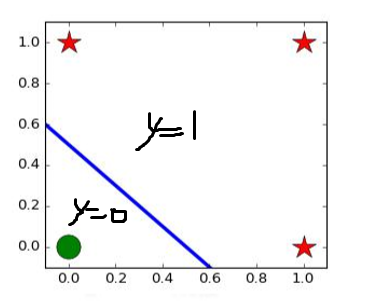$x_1$ $x_2$ $h_\theta(x)$
0 0 0
0 1 1
1 0 1
1 1 1
• 数据为线性不可分时：
一条直线无法将数据进行划分，如下图：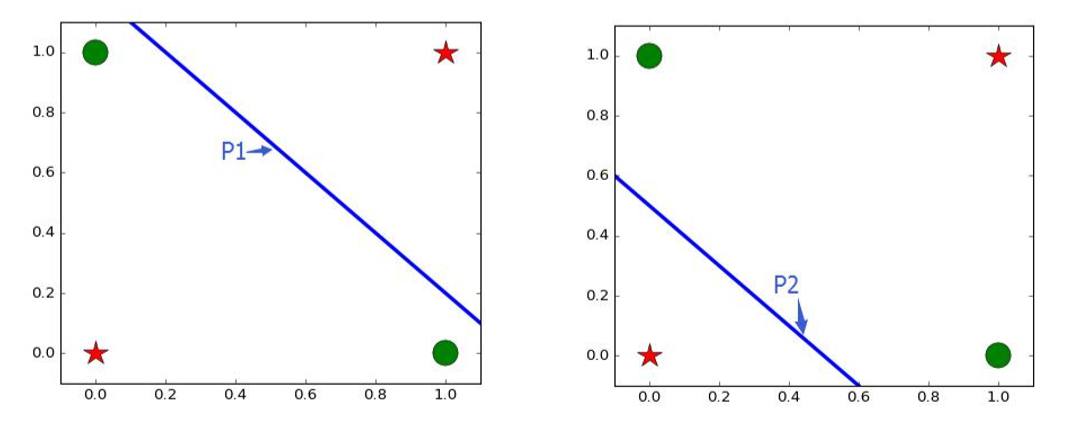对于上面数据需要多个感知器，意思就是多条直线，多重感知机(简单的神经网络)！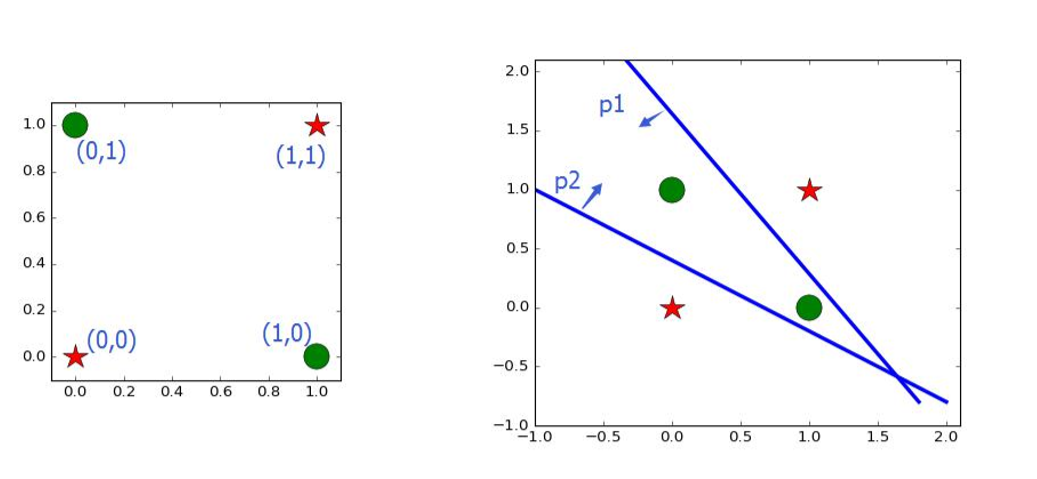结构如下图：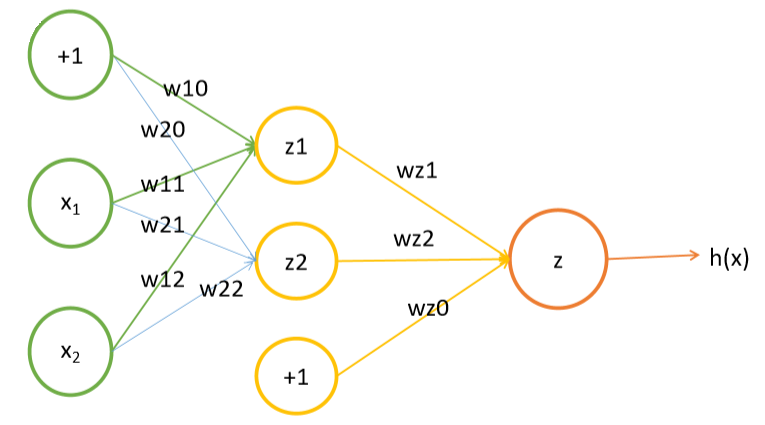$w_1=(-3,2,2)\\ w_2=(-1,2,2)\\ w_z=(1,3,-3)$$h_w(z)=h(Wx)=\begin{cases}0,z<0\\1,z\geq0\end{cases}$
$x_1$ $x_2$ $z_1$ $z_2$ $h(x)$
0 0 0 0 1
0 1 0 1 0
1 0 0 1 0
1 1 1 1 1

# 神经网络-基本架构

## 简单的神经网络

$f(h)=\begin{cases}0,if \quad h<0\\1,if \quad z\geq0\end{cases}$$h=\sum_iw_ix_i+b$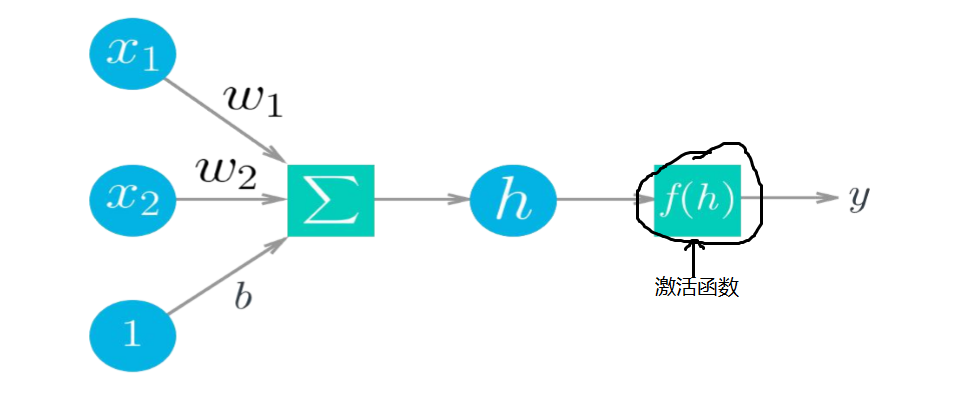## 感知器 and S型神经元

• 感知器这种网络很难学习非线性的问题，因为激活函数是阶跃函数（不可导，不可积，不可微），所以使用sigmoid函数（可导，可积，可微来代替它，从而S型神经网络可以解决这一问题。

• 感知器模型中，我们可以将单个神经元的计算过程看成下列两个步骤： 先

• 计算权重w和输入值x以及偏置项b之间的线性结果值z：z=wx+b
• 然后对结果值z进行一个数据的**sign函数(阶跃函数)**转换，得到一个离散的0/1值: y=int((sign(z)+1)/2)
• 输入：x1、x2、x3和截距+1

• 输出：函数hw,b(x)，其中w权重和b偏置项是参数

• S型神经元中，和感知器神经元的区别在于： 对于结果值z的转换，采用的不是sign函数进行转换，是采用平滑类型的函数进行转换，让输出的结果值y最终是一个连续的，S型神经元转指使用的是sigmoid函数

• 输入：$x_1、x_2、x_3$和截距+1
• 输出：函数$h_{w,b}(x)$，其中w权重和b偏置项是参数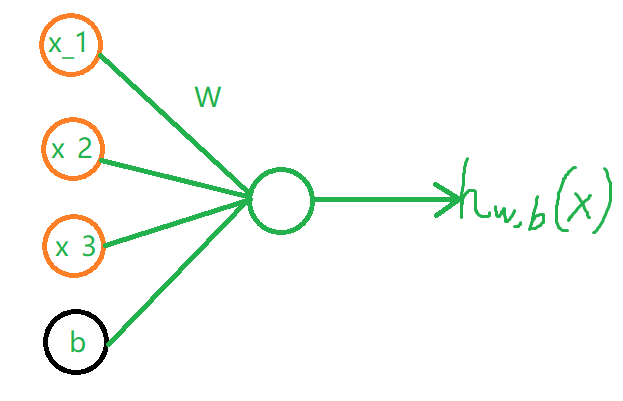$h_{w,b}(x)=f(W^Tx,b)=f(\sum^3_{i=1}W_ix_i+b)$

## 激活函数

tanh(双曲正切函数):$tanh(z)=f(z)=\frac{e^z-z^{-z}}{e^z+z^{-z}}$$f'(z)=1-(f(z))^2$
sigmoid(逻辑 回归函数)：导数介于（0，0.25）之间$sigmiod(z)=\frac{1}{1+e^{-z}}$$f'(z)=f(z)(1-f(z))$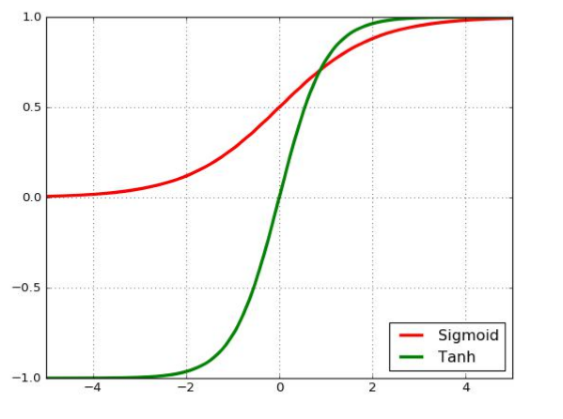• 作用： 激活函数的主要作用是使模型获得非线性的能力。如果没有激活函数，那么该网络仅能够表达线性映射，此时即便有再多的隐藏层，其整个网络跟单层神经网 络也是等价的。因此也可以认为，只有加入了激活函数之后，深度神经网络才具 备了分层的非线性映射学习能力。 激活函数的主要特性是：可微性、单调性、输出值的范围

# 神经网络(DNN)

• 神经网络主要由三个组成部分

• 第一个是架构（architecture）或称为拓扑结构 （topology），描述神经元的层次与连接神经元的结构。
• 第二个组成部分是神 经网络使用的激励/激活函数。
• 第三个组成部分是找出最优权重值的学习算法。
• 神经网络主要分为两种类型

• 前馈神经网络(Feedforward Neural Networks) 是最常用的神经网络类型（如FC CNN），一般定义为有向无环图，信号只能沿 着最终输出的那个方向传播。
• 另外一个是反馈神经网络(Feedback Neural Networks)，也称为递归神经网络(Recurent Neural Networks)，也就是网络中 环。
• 神经网络的一般结构是由输入层、隐藏层(神经元)、输出层构成的。隐藏层可以是1层或者多层叠加，层与层之间是相互连接的

## 浅层神经网络结构

• 添加少量隐层的神经网络就叫做浅层神经网络；也叫作传统神经网络，一般为2 隐层的神经网络(超过两隐层的话，效果会差很多)
下图为全连接（前馈神经网络）一般应用于分类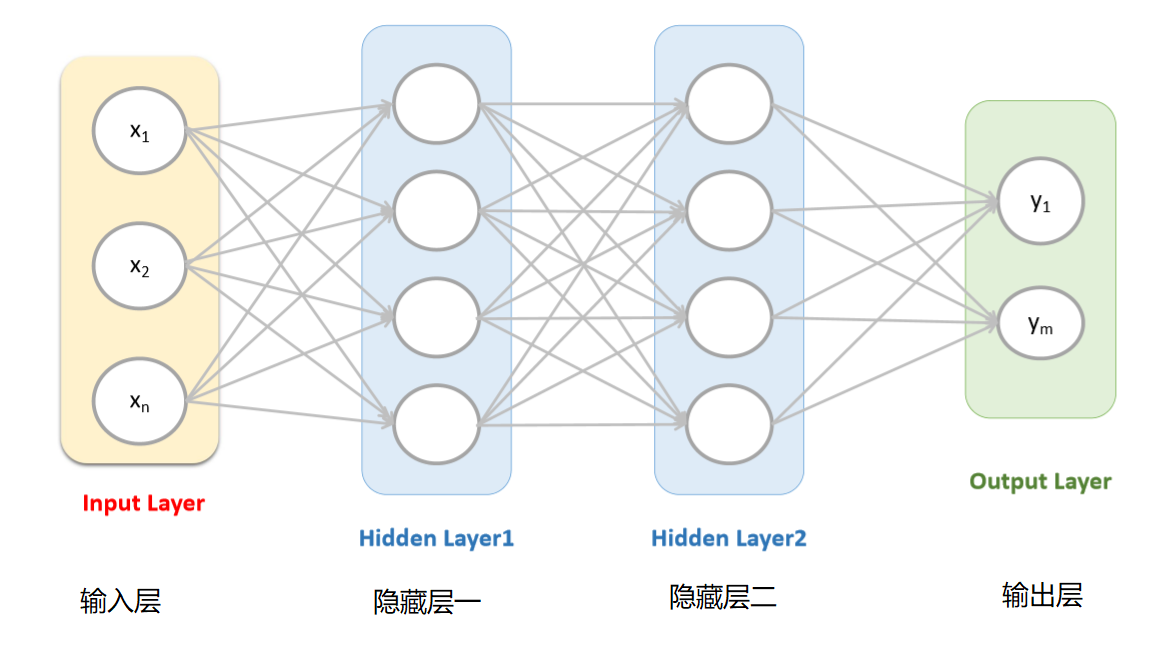## 深度的神经网络

• 增多中间层(隐层)的神经网络就叫做深度神经网络(DNN)；可以认为深度学习是 神经网络的一个发展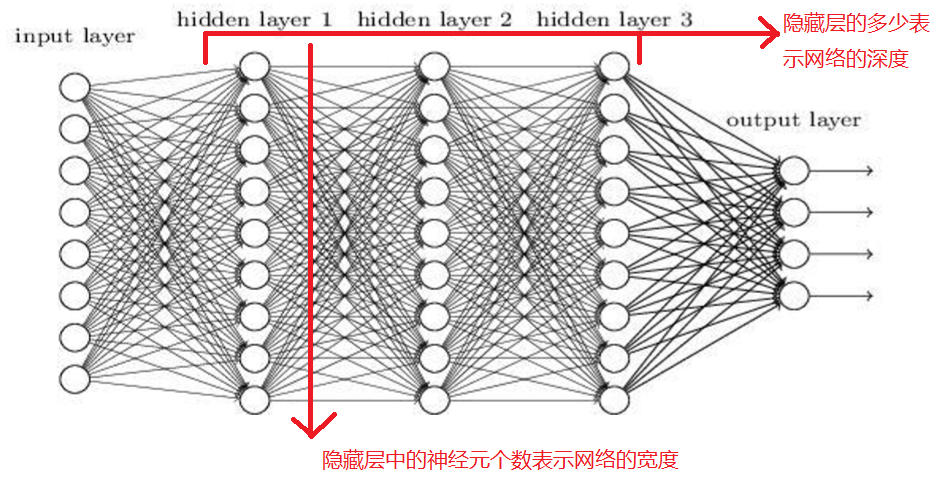## 神经网络之非线性可分

• 对线性分类器的与(and)和或(or)的组合可以完成非线性可分的问题；
• 即通过多层的神经网络中加入激活函数的方式可以解决非线性可分的问题。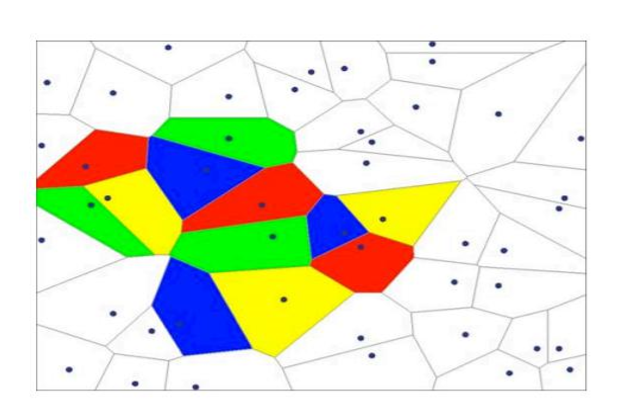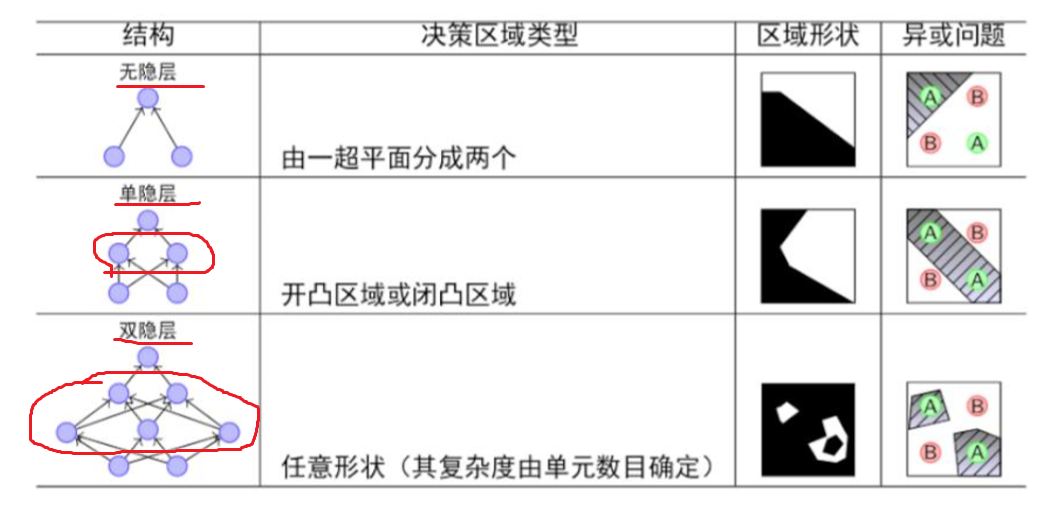## 神经网络之过拟合

• 理论上来讲，单隐层的神经网络可以逼近任何连续函数（只要隐层的神经元 个数足够的多<一个神经元将数据集分为两类>）
• 虽然从数学表达上来讲，效果一样，但是在网络工程效果中，多隐层的神经 网络效果要比单隐层的神经网络效果好
• 对于一些分类的问题来讲，三层的神经网络效果优于两层的神经网络，但是 如果把层次不断增加(4,5,6,7…)，对于最终的效果不会产生太大的变化
• 提升隐层层数或者神经元个数，神经网络的“容量”会变大，那么空间表达 能力会变强（模型的拟合能力变强），从而有可能导致过拟合的问题
• 对于视频/图片识别/文本等问题，传统的神经网络(全连接神经网络)不太适 合

# 反向传播神经网络(BPNN)

## 梯度下降

• 评判预测有多差的指标（即误差error）：较通 用的指标:SSE(误差平方和)，公式为：$E=\frac{1}{2}\sum_{\mu}\sum_{j}\left[y^\mu_j-\hat{y}^\mu_j\right]^2$

• 交叉熵损失函数为（二分类）：$E=-\frac{1}{m}(y\ln\hat{y}+(1-y)\ln(1-\hat{y}))$

• 梯度：就是斜率或者斜度的另一个称呼。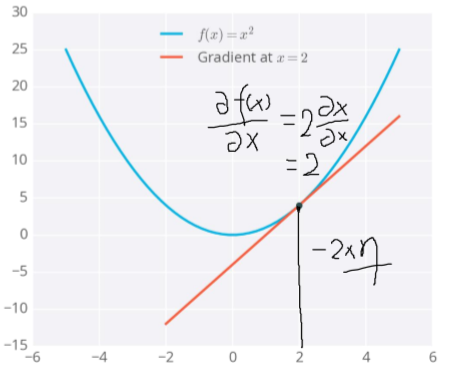• 下图是一个拥有两个输入的神经网络误差 示例，相应的，它有两个权重。可以 看成 一个地形图，同一条线代表相同的误差， 较深的线对应较大的误差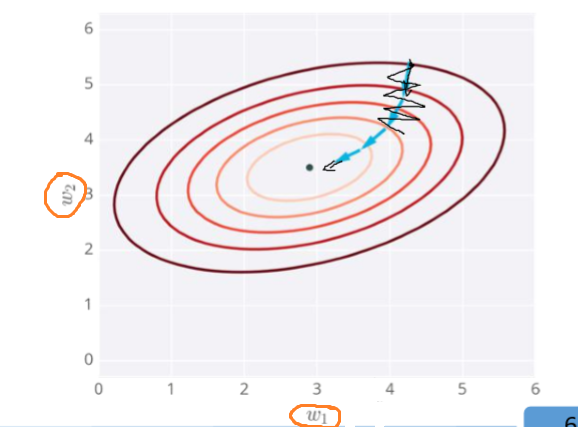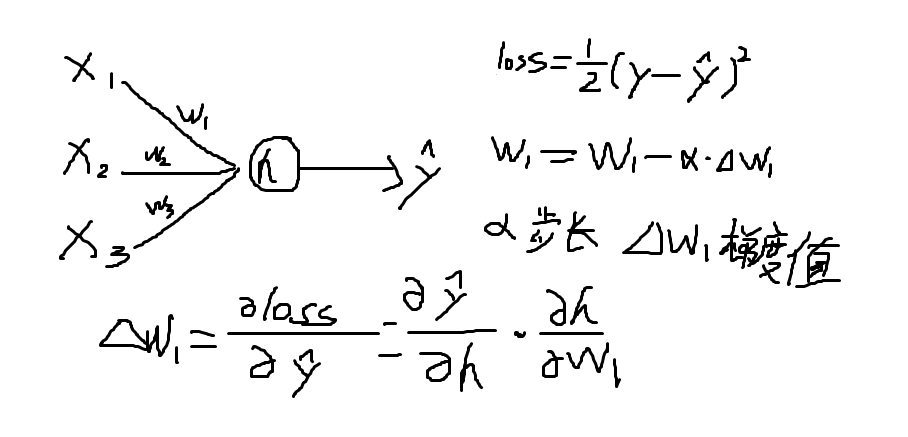## 反向传播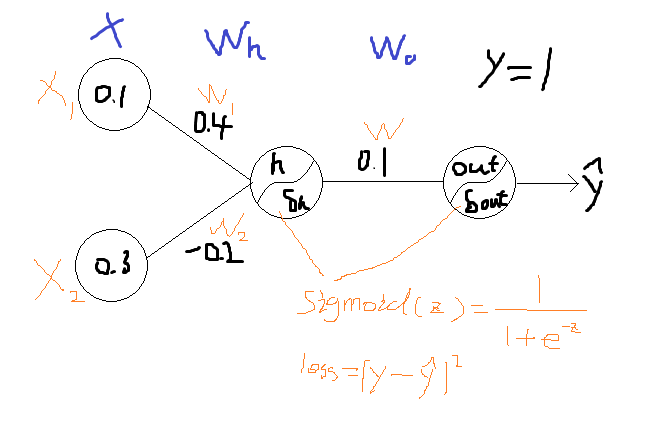### 正向传播过程：

$h=X\cdot W_h^T=\sum_{i=1}^2x_iw_i \\\delta_h=sigmoid(h)\\out=\delta_h\cdot W_o\\\delta_{out}=sigmoid(out)$

\begin{aligned}h&=(x_1\cdot w_1)+(x_2\cdot w_2) \\&=0.1*0.4+0.3*(-0.2) \\&=-0.02 \end{aligned}\tag 1
\begin{aligned}\delta_h&=\frac{1}{1+e^{-h}} \\&=\frac{1}{1+e^{0.02}} \\&=0.495 \end{aligned}\tag 2
\begin{aligned}out&=\delta_h\cdot w \\&=0.495*0.1 \\&=0.0495 \end{aligned}\tag 3
\begin{aligned}\delta_{out}&=\frac{1}{1+e^{-out}} \\&=\frac{1}{1+e^{-0.0495}} \\&=0.512 \end{aligned}\tag 4
$\delta_{out}=\hat{y}$

$loss=\frac{1}{2}(y-\hat{y})^2$

### 反向传播过程：

$\delta_w=\frac{\partial loss}{\partial \delta_w}\Rightarrow\frac{\partial loss}{\partial \hat{y}}\cdot \frac{\partial \hat{y}}{\partial out}\cdot\frac{\partial out}{\partial w}$$\delta_{w_1}=\frac{\partial loss}{\partial \delta_{w_1}}\Rightarrow\frac{\partial loss}{\partial \hat{y}}\cdot \frac{\partial \hat{y}}{\partial out}\cdot\frac{\partial out}{\partial \delta _h}\cdot \frac{\partial \delta _h}{\partial h}\cdot\frac{\partial h}{\partial w_1}$$\delta_{w_2}=\frac{\partial loss}{\partial \delta_{w_2}}\Rightarrow\frac{\partial loss}{\partial \hat{y}}\cdot \frac{\partial \hat{y}}{\partial out}\cdot\frac{\partial out}{\partial \delta _h}\cdot \frac{\partial \delta _h}{\partial h}\cdot\frac{\partial h}{\partial w_2}$

# DNN中的问题

• 一般来讲，可以通过增加神经元和网络层次来提升神经网络的学习能力，使其得到的模型更加能够符合数据的分布场景；但是实际应用场景中，神经网络的层次一般情况不会太大，因为太深的层次有可能产生一些求解的问题
• 在DNN的求解中有可能存在两个问题：梯度消失梯度爆炸；我们在求解梯度的时候会使用到链式求导法则，实际上就是一系列的连乘，如果每一层都 小于1的话，则梯度越往前乘越小，导致梯度消失，而如果连乘的数字在每层 都是大于1的，则梯度越往前乘越大，导致梯度爆炸。

# 为什么说神经网络是端到端的网络?

• 优点：

• 通过缩减人工预处理和后续处理，尽可能使模型从原始输入到最终输出，给模型更多可以根据数据自动调节的空间，增加模型的整体契合度。
• 缺点

• 它可能需要大量的数据。要直接学到这个𝑥到𝑦的映射，你可能需要大量(𝑥, 𝑦)数据。
• 它排除了可能有用的手工设计组件。

# softmax分类器

softmax回归和线性回归一样将输入特征与权重做线性叠加。与线性回归的一个主要不同在于，softmax回归的输出值个数等于标签里的类别数。 下图是用神经网络描绘了softmax回归，也是一个单层神经网络，由于每个输出$o_1,o_2,o_3$的计算都要依赖于所有的输入 $x_1,x_2,x_3,x_4$，softmax回归的输出层也是一个全连接层。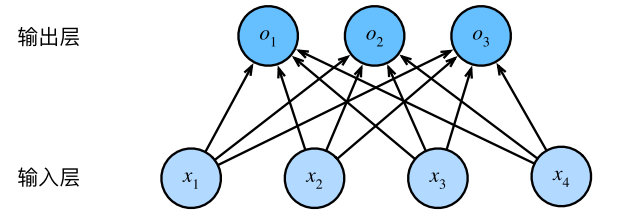$o_1=x_1w_{11}+x_2w_{21}+x_3w_{31}+x_4w_{41} \\o_2=x_1w_{12}+x_2w_{22}+x_3w_{32}+x_4w_{42}\\o_3=x_1w_{13}+x_2w_{23}+x_3w_{33}+x_4w_{43}\\o_4=x_1w_{14}+x_2w_{24}+x_3w_{34}+x_4w_{44}$

## softmax的计算

• 由于输出层的输出值的范围不确定，我们难以直观上判断这些值得意义。
• 由于真实标签是离散值，这些离散值与不确定范围的输出值之间的误差难以衡量。

softmax运算解决了以上两个问题。它通过下面的公式将输出值变换成值为正并且和为1的概率分布：$softmax(o_i)=\frac{exp(o_i)}{\sum^n_{i=1}exp(o_i)}$

# 交叉熵损失函数

• 我们已经知道，softmax运算将输出变换成一个合法的类别预测分布。实际上，真实标签也可以用类别分布表达：

• 对于样本i，我们构造向量$y^{(i)}\in_R^q$，使其第$y^{(i)}$个元素为1，其余为0。这样我们的训练目标可以设为使预测概率分布 $\hat{y}^{(i)}$尽可能接近真实的标签概率$y^{(i)}$

• 想要预测分类结果正确，我们其实并不需要预测概率完全等于标签概率，而平方损失则过于严格。改善这个问题的一个方法是使用更适合衡量两个概率分布差异的测量函数。其中，交叉熵(cross entropy)是一个常用的衡量方法：$H(y^{(i)},\hat{y}^{(i)})=-\sum_{j=1}^qy^{(i)}_j\log\hat{y}^{(i)}_j=-\log\hat{y}^{(i)}_{y^{(i)}}$其中带下标的$y^{(i)}_j$是向量$y^{(i)}$中非 0 即 1 的元素。也就是说，交叉熵只关心对正确类别的预测概率，因为只要其值足够大，就可以确保分类结果正确。即最小化交叉熵损失函数等价于最大化训练数据集所有标签类别的联合预测概率。

2017-08-03 17:37:47 Harriet666 阅读数 200
• ###### MATLAB图像处理

全面系统的学习MATLAB在图像处理中的应用

20258 人正在学习 去看看 魏伟
PDF Extractor SDK是一款可以转换PDF为文本，CSV，XML，从PDF文件中提取图像和信息的多功能工具包。

PDF Extractor SDK v8.6.0.2911更新内容：
• XMLExtractor，JSONExtractor，HTMLExtractor：添加了KeepOriginalFontNames属性。
• TextComparer：添加GetChanges（）方法以获得比较结果，方便进行程序化分析。
• DocumentRotator：现在可以指定要旋转的页面。
• TextExractor.ExtractColumnByColumn属性现在影响Find（）方法。
• 修复SearchResult元素中的字体名称。
• 修复对比度预处理过滤器。
• 修复下标和上标文本对象与正常文本合并的问题。
• 其他小修改和改进。

2019-06-24 11:00:03 weixin_34082854 阅读数 34
• ###### MATLAB图像处理

全面系统的学习MATLAB在图像处理中的应用

20258 人正在学习 去看看 魏伟
1.  返回顶部按钮
2.  预加载图像
3.  检查图像是否加载
4.  自动修复破坏的图像
5.  悬停切换类
6.  禁用输入字段
7.  停止加载链接
8.  切换淡入/幻灯片
9.  简单的手风琴
10.  让两个div高度相同
11.  在新标签页/窗口打开外部链接
12.  通过文本查找元素
13.  在改变visibility时触发
14.  AJAX调用错误处理
15.  链式插件调用

// Back to top$('.top').click(function (e) { e.preventDefault();$('html, body').animate({scrollTop: 0}, 800);});

<!-- Create an anchor tag -->

$.preloadImages = function () { for (var i = 0; i < arguments.length; i++) {$('<img>').attr('src', arguments[i]);
}
};

$.preloadImages('img/hover-on.png', 'img/hover-off.png'); 检查图像是否加载 有时为了继续脚本，你可能需要检查图像是否全部加载完毕：$('img').load(function () {
});

$('img').on('error', function () { if(!$(this).hasClass('broken-image')) {
$(this).prop('src', 'img/broken.png').addClass('broken-image'); } }); 即使没有任何断掉的链接，加上这一段代码也不会让你有任何损失。 悬停切换类 假设你希望当用户将鼠标悬停在可点击的元素上时，它会改变颜色。那么你可以在用户悬停的时候添加类到元素中，反之则删除类：$('.btn').hover(function () {
$(this).addClass('hover'); }, function () {$(this).removeClass('hover');
});

$('.btn').hover(function () {$(this).toggleClass('hover');
});

$('input[type="submit"]').prop('disabled', true); 然后你只需要运行输入的 prop 方法就可以了，不过 disabled 的值要设置为false：$('input[type="submit"]').prop('disabled', false);

$('a.no-link').click(function (e) { e.preventDefault(); }); 淡入/滑动切换 滑动和淡入都是我们用jQuery做动画的时候大量运用的东西。如果你只是想在用户点击之后展示一个元素的话，那么用 fadeIn 和 slideDown 方法就很完美。但是，如果你想要元素在第一次点击的时候出现，然后在第二次点击的时候消失的话，那么可以试试下面的代码： // Fade$('.btn').click(function () {
$('.element').fadeToggle('slow'); }); // Toggle$('.btn').click(function () {
$('.element').slideToggle('slow'); }); 简单的手风琴 这是一个可快速生成手风琴的简单方法： // Close all panels$('#accordion').find('.content').hide();

// Accordion
$('#accordion').find('.accordion-header').click(function () { var next =$(this).next();
next.slideToggle('fast');
$('.content').not(next).slideUp('fast'); return false; }); 通过添加这个脚本，你真正需要做的仅仅是在页面上添加必要的HTML元素，这样它就可以运行工作了。 让两个div高度相同 有时候，你需要让两个div无论包含什么内容都拥有相同的高度：$('.div').css('min-height', $('.main-div').height()); 设置 min-height ，这意味着它可以比主div大但绝对不能比主div小。不过，还有一种更灵活的方法是遍历一组元素，然后将高度设置为最高的那个元素的高度： var$columns = $('.column'); var height = 0;$columns.each(function () {
if ($(this).height() > height) { height =$(this).height();
}
});
$columns.height(height); 如果你希望所有列的高度相同： var$rows = $('.same-height-columns');$rows.each(function () {
$(this).find('.column').height($(this).height());
});

$('a[href^="http"]').attr('target', '_blank');$('a[href^="//"]').attr('target', '_blank');
$('a[href^="' + window.location.origin + '"]').attr('target', '_self'); 注意：window.location.origin 在IE10中无效。修复的时候要小心这个问题。 通过文本查找元素 通过使用jQuery中的 contains() 选择器，你可以找到元素内容的文本。如果文本不存在，那就隐藏该元素： var search =$('#search').val();
$('div:not(:contains("' + search + '"))').hide(); 在改变Visibility时触发 当用户不再关注某个tab，或重新聚焦原来的那个tab上时，触发JavaScript：$(document).on('visibilitychange', function (e) {
if (e.target.visibilityState === "visible") {
console.log('Tab is now in view!');
} else if (e.target.visibilityState === "hidden") {
console.log('Tab is now hidden!');
}
});

AJAX调用错误处理

$(document).ajaxError(function (e, xhr, settings, error) { console.log(error); }); 链式插件调用 jQuery允许“链式”插件的方法调用，以减轻反复查询DOM并创建多个jQuery对象的过程。比方说，下面的代码片段代表了你的插件方法调用：$('#elem').show();
$('#elem').html('bla');$('#elem').otherStuff();

$('#elem') .show() .html('bla') .otherStuff(); 还有一种方法是在（前缀$）变量中高速缓存元素：

var $elem =$('#elem');
$elem.hide();$elem.html('bla');
$elem.otherStuff(); 链式和高速缓存的方法都是jQuery中可以让代码变得更短和更快的代最佳做法。 转载于:https://www.cnblogs.com/Alenliu/p/5158124.html 图像处理 文本图像修复 相关内容 2017-02-07 10:41:00 weixin_30845171 阅读数 6 • ###### MATLAB图像处理 全面系统的学习MATLAB在图像处理中的应用 20258 人正在学习 去看看 魏伟 下面这些简单的小技巧能够帮助你玩转jQuery。 • 返回顶部按钮 • 预加载图像 • 检查图像是否加载 • 自动修复破坏的图像 • 悬停切换类 • 禁用输入字段 • 停止加载链接 • 切换淡入/幻灯片 • 简单的手风琴 • 让两个div高度相同 • 在新标签页/窗口打开外部链接 • 通过文本查找元素 • 在改变Visibility时触发 • AJAX调用错误处理 • 链式插件调用## 返回顶部按钮 通过使用jQuery中的animate 和scrollTop 方法，不用插件就可以创建一个滚动到顶部的简单动画： // Back to top$('.top').click(function (e) {
e.preventDefault();
$('html, body').animate({scrollTop: 0}, 800); }); <!-- Create an anchor tag --> <a class="top" href="#">Back to top</a> 改变scrollTop 的值可以更改你想要放置滚动条的位置。所有你真正需要做的是在800毫秒的时间内设置文档主体的动画，直到它滚动到文档的顶部。 注：小心scrollTop的一些错误行为。 ## 预加载图像 如果你的网页要使用大量开始不可见的（例如，悬停的）图像，那么可以预加载这些图像： $.preloadImages = function () { for (var i = 0; i < arguments.length; i++) {
$('<img>').attr('src', arguments[i]); } };$.preloadImages('img/hover-on.png', 'img/hover-off.png');

}
});

## 悬停切换类

$('.btn').hover(function () {$(this).addClass('hover');
}, function () {
$(this).removeClass('hover'); }); 你只需要添加必要的CSS即可。更简单的方法是使用toggleClass 方法： $('.btn').hover(function () {

$('input[type="submit"]').prop('disabled', false); ## 停止加载链接 有时候，你既不需要链接到某个特定的网页，也不想要重新加载页面——你可能希望链接做点别的事情，例如说触发一些其他脚本。这就要在阻止默认动作上做文章了： $('a.no-link').click(function (e) {
e.preventDefault();
});

## 淡入/滑动切换

// Fade $('.btn').click(function () {$('.element').fadeToggle('slow');
}); // Toggle $('.btn').click(function () {$('.element').slideToggle('slow');
});

## 简单的手风琴

// Close all panels $('#accordion').find('.content').hide(); // Accordion$('#accordion').find('.accordion-header').click(function () { var next = $(this).next(); next.slideToggle('fast');$('.content').not(next).slideUp('fast'); return false;
});

## 让两个div高度相同

$('.div').css('min-height',$('.main-div').height());

var $columns =$('.column'); var height = 0;
$columns.each(function () { if ($(this).height() > height) {
height = $(this).height(); } });$columns.height(height);

var $rows =$('.same-height-columns');
$rows.each(function () {$(this).find('.column').height($(this).height()); }); ## 在新标签页/窗口打开外部链接 在一个新的浏览器tab或窗口中打开外部链接，并确保同一个来源的链接能在同一个tab或者窗口中打开： $('a[href^="http"]').attr('target', '_blank');
$('a[href^="//"]').attr('target', '_blank');$('a[href^="' + window.location.origin + '"]').attr('target', '_self');

## 通过文本查找元素

var search = $('#search').val();$('div:not(:contains("' + search + '"))').hide();

});

## 链式插件调用

jQuery允许“链式”插件的方法调用，以减轻反复查询DOM并创建多个jQuery对象的过程。比方说，下面的代码片段代表了你的插件方法调用：

$('#elem').show();$('#elem').html('bla');
$('#elem').otherStuff(); 通过使用链式，可以大大改善： $('#elem')
.show()
.html('bla')
.otherStuff();

$elem.html('bla');$elem.otherStuff();